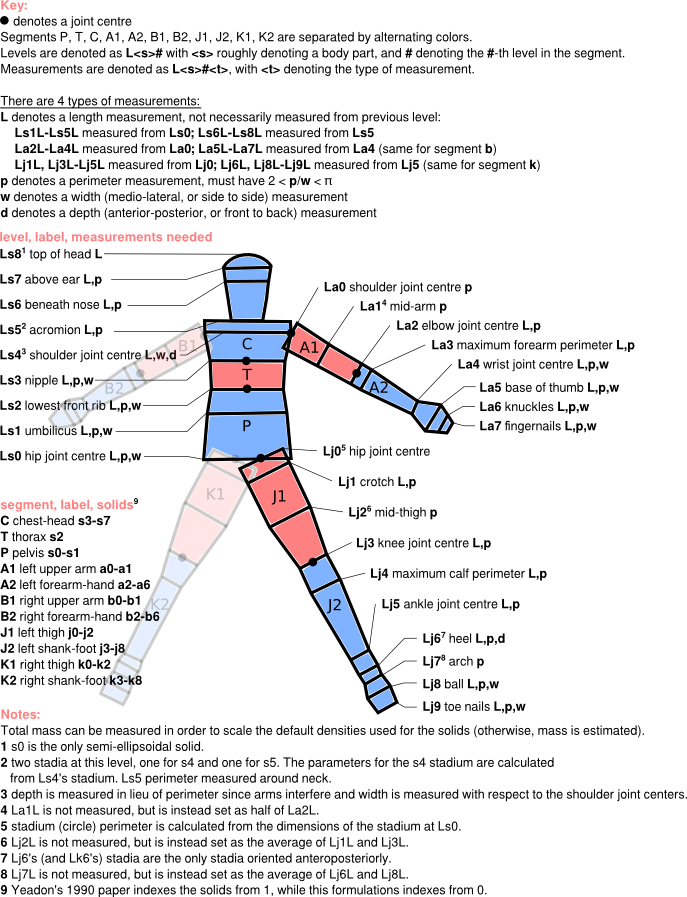# Measurements¶

Here we describe the measurements that need to be taken to define a human with this model, and we provide some guidance for taking those measurements and getting them into the code.

## The stadium shape and the stadium solid¶

The human is composed of 11 rigid body segments. Each segment is defined as a loft across a number of 2D parallel stadium shapes, which are defined below. In one case, a segment contains a semi-ellipsoid solid. The model is customized to an individual via 95 anthropomorphic measurements to define the stadia and the distances between them.

A stadium shape, show in the figure below, can be defined via any of the following 4 sets of 2 parameters:

• a radiusand thickness,
• a perimeterand widthalong the stadium’s longitudinal axis
• a perimeterand a depth.
• a depthand a width.A circle can be defined by a stadium whose thickness is zero,.

Stadium solids are defined by two parallel stadia, as well as the heightof the solid between the two stadia (i.e., a loft between the two stadium cross sections).

## Specification of all measurements¶

The figure below specifies all 95 measurements.

To define the stadium solids that make up the human model, one takes the measurements outlined here. The measurements consist of lengths(different from heights), perimeters, widths, and depths.

By measuring the parameters that define the stadia (also called levels), and the distance between these stadia, we define 39 stadium solids. Each stadium is shared by two stadium solids, except for the stadia at the end of the hands and feet. In general, the stadia are defined by measuring perimeter and width, since these are easier to measure. There are a few exceptions though, and these are described further down the page.It is lengths, not heights, that you measure off the subjects. That is, the length measurements are sums of heights, not the individual heights of the stadium solids. For example, The “length” for the Ls5 acromion level is measured from Ls0, the hip joint centre, not from Ls4. The figure above lists the level from which the length for other levels are measured.

## Scaling densities via a measured mass¶

The mass of the model is estimated from the measurements described above, along with densities for the various segments taken from the literature. In the case that you also measure the mass of the individual being modeled, it is possible to scale the densities so that the total mass of the human is that which you have measured. See Usage for a brief explanation on how to do this.

## Exceptions to the general measurement practice¶

There are a number of exceptions to the general scheme of measurements required by the model.

• Length exceptions: Lengths to arm level 1 and leg levels 2 and 7 are not measured. The length from La0 to La1 (or Lb0 to Lb2) is set internally as half the length from La0 to La2 (or Lb0 to Lb2). The lengths to leg levels 2 and 7 are calculated as averages of the two lengths around leg levels 2 and 7. Thus, perimeters, etc. at these levels should be measured halfway between the surrounding levels (i.e., perimeter of the La1 stadium is measured at the point in in the arm halfway between La0 and La2).
• Levels that are circles (zero-thickness stadia): Arm levels 0-3 (the first four arm levels) and leg levels 0-5 and 7 (the first six and the arch). For these, only a perimeter measurement is required (no width or depth is measured).
• Depth measurements: As far as measurements are concerned, the only difference between a depth and a width is that a depth is measured anterior to posterior (front to back), while widths are measured medio-laterally (side to side) when the subject is in the configuration as drawn in the diagram above. Depths are measured at the Ls5 acromion, and the Lj6, Lk6 heel.
• The neck: The base of the neck, which is also located at level Ls5, acromion, is modeled as circular. Its radius is set internally from the acromion perimeter measurement. This means that the acromion perimeter should be measured about the base of the neck.

## Getting measurements into the model¶

There are two options for getting measurements into the model:

• Use the meastemplate.txt input text file in the misc/ directory, or `here`, to define all measurements. The file uses the YAML syntax. This syntax allows you to treat the input file as a Python script in which you simply define a number of variables. See comments within the file for further details.
• Provide a python dictionary, containing all the appropriate fields, to the `yeadon.human.Human` constructor. You can obtain a sample dictionary from the variable `yeadon.human.Human.meas`. The keys for the dictionary are the names of the variables in the meastemplate.txt file, as strings.

Internally, the package uses units of meters for the measurements. However, it may be that you have worked with different units in gathering the measurements. In the case that you are using an input text file to specify measurements (i.e., meastemplate.txt), you can define the measurements using the units you desire, and the package will perform the unit conversion for you. This is done by providing a value for the variable `measurementconversionfactor` in the text file, as shown in meastemplate.txt. This is a number that converts the units of your measurements into meters. For example, if you took measurements in millimeters, you should give this variable the value 0.001. If you are providing measurements via a dictionary, the measurements must be in units of meters.

## Sample measurement files¶

Here are measurement data files for three people we measured: